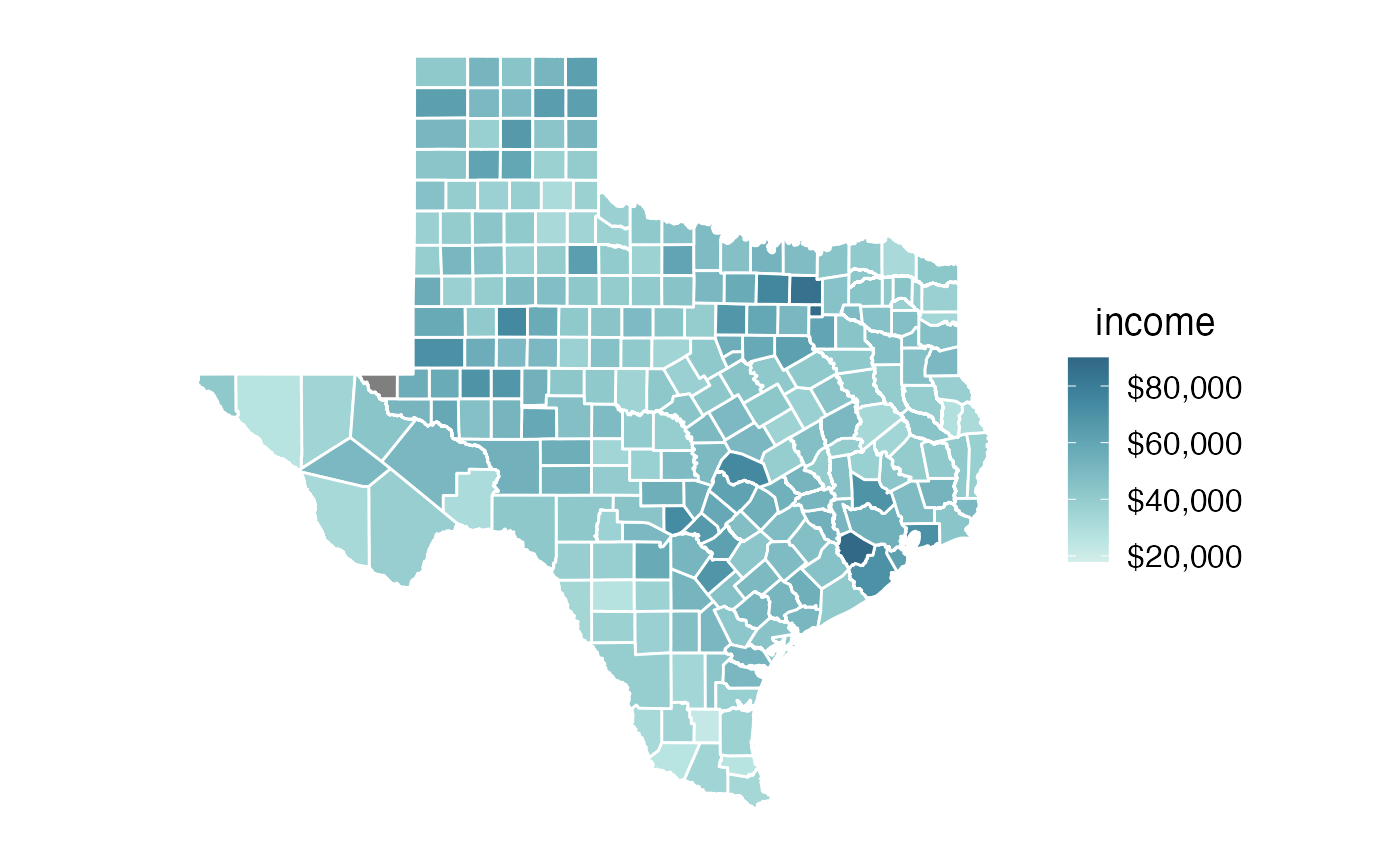Median income in Texas counties, from the 2015 five-year American Community Survey.

texas_income

## Format

An object of class sf (inherits from data.frame) with 254 rows and 6 columns.

## Examples

library(tidyverse)
library(sf)
#> Linking to GEOS 3.8.1, GDAL 3.1.4, PROJ 6.3.1library(cowplot)
library(colorspace)

# B19013_001: Median household income in the past 12 months (in 2015 Inflation-adjusted dollars)

texas_income %>%
ggplot(aes(fill = estimate)) +
geom_sf(color = "white") +
coord_sf(datum = NA) +
theme_map() +
scale_fill_continuous_sequential(
palette = "Teal", rev = TRUE,
na.value = "grey50", end = 0.9,
name = "income",
limits = c(18000, 90000),
breaks = 20000*c(1:4),
labels = c("$20,000", "$40,000", "$60,000", "$80,000")
) +
theme(
legend.title.align = 0.5,
legend.text.align = 0
)